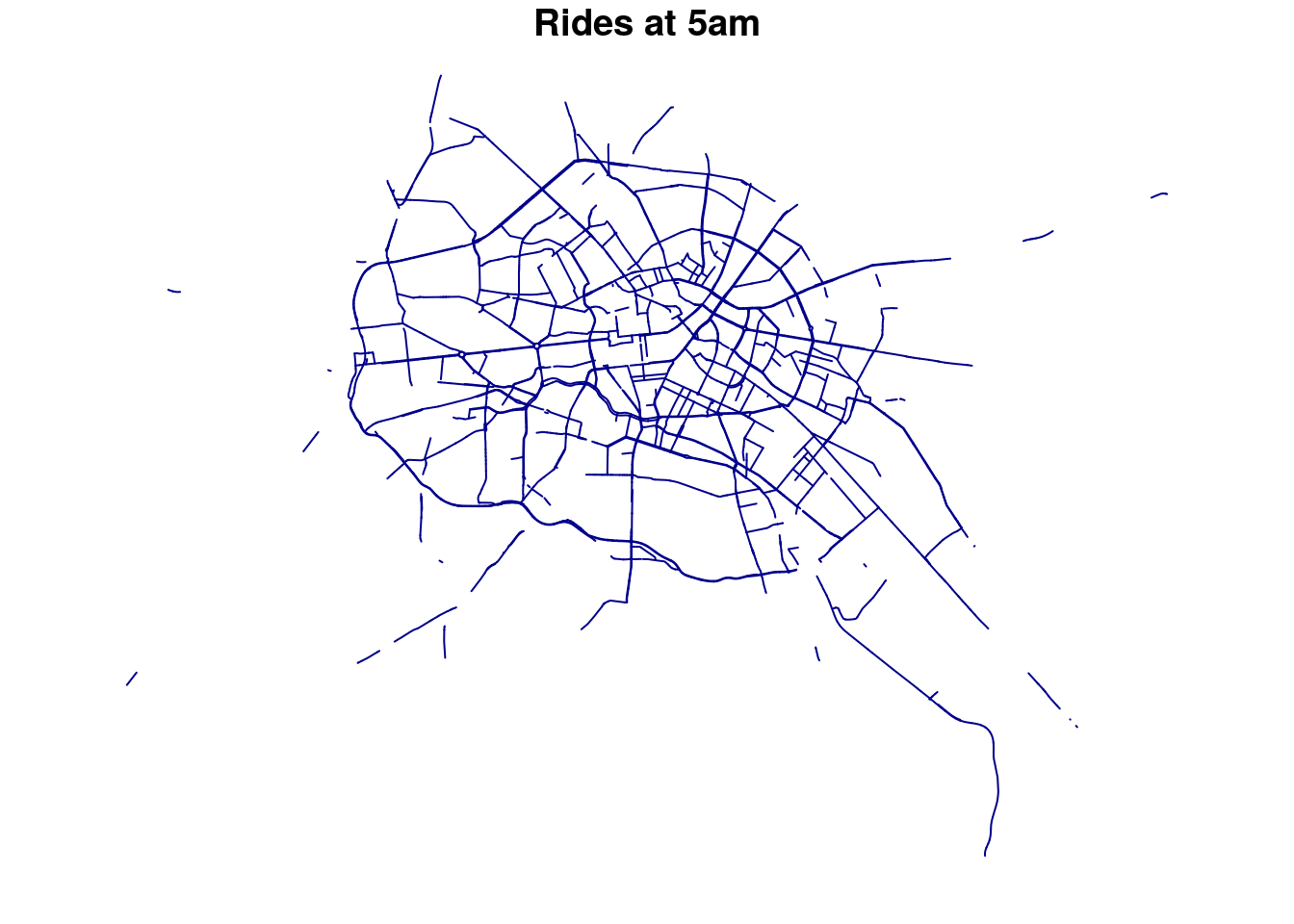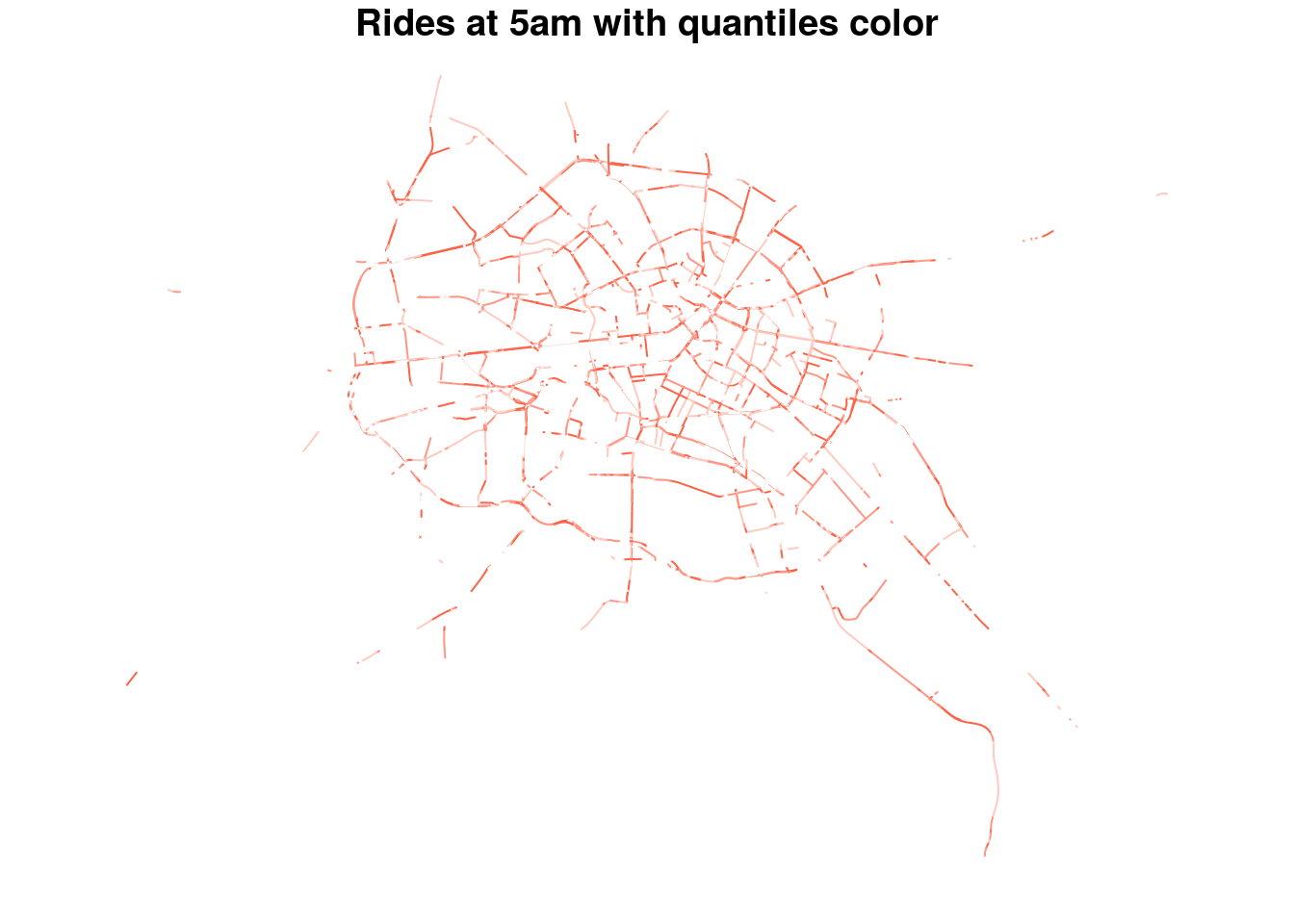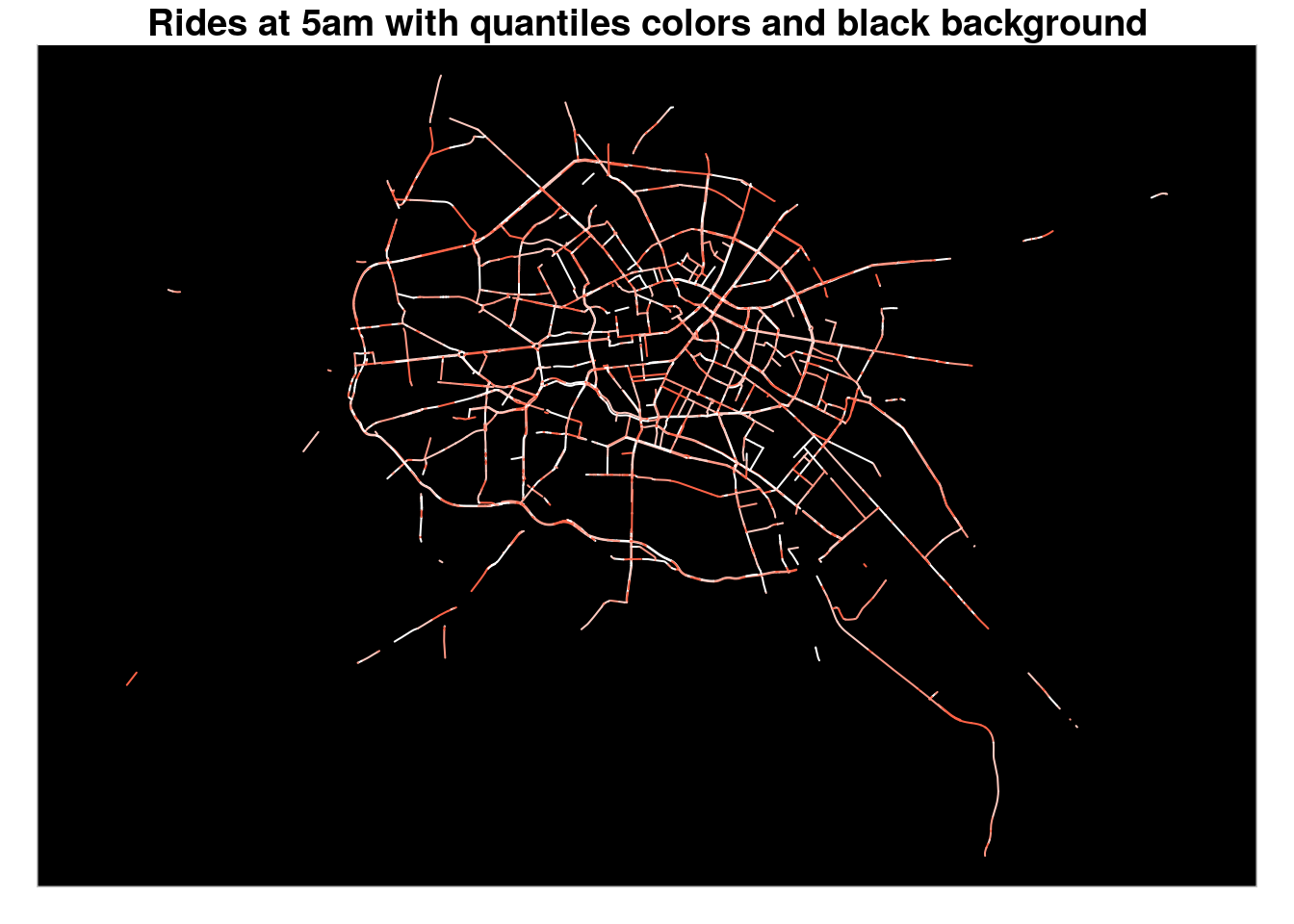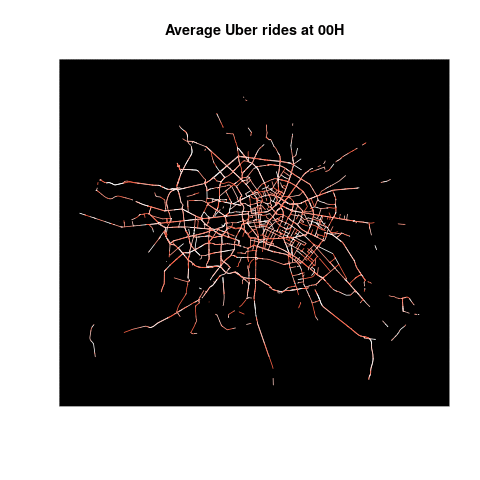# Chapter 6 Visualizations with Simple Features

Now that we have an idea of the content of our dataset, we can focus on its geospatial aspect.

## 6.1 Merge the data (again)

We first merge our `DT.max.speed.per.way.hour` table with the OSM shapefile :

``````df.Uber <- as.data.frame(DT.max.speed.per.way.hour[,name:=NULL])
OSM_sf.with.Uber <-  merge(OSM_sf,df.Uber,by.x='osm_id',by.y='osm_id',all.y=T)``````

Note that in order to merge both, we must convert our data.table `DT.max.speed.per.way.hour` to a data.frame.

Can we resist to look at the Uber data for the streets (ways) that are defined as ‘pedestrian’ ?

No.

``OSM_sf.with.Uber %>% filter(fclass=='pedestrians')``
``````## Simple feature collection with 0 features and 16 fields
## bbox:           xmin: NA ymin: NA xmax: NA ymax: NA
## epsg (SRID):    4326
## proj4string:    +proj=longlat +datum=WGS84 +no_defs
##   osm_id          code            fclass          name
##   ref             oneway          maxspeed.x      layer
##   bridge          tunnel          hour            total_rows
##  ratio.over      speed_kph_mean  maxspeed.y      speed_minus_max
##  geometry
## <0 rows> (or 0-length row.names)``````

A very clean dataset indeed, not a single row for ways defined by OSM as pedestrian.

How about the other types of ways ? We aggregate the data per `class` and compute the mean of the speed limit infrigment ratio :

``````DT.OSM.max.speed <-  as.data.table(OSM_sf.with.Uber)
DT.OSM.max.speed[,.(total_fclass=.N, mean_ratio.over =round(mean(ratio.over),2)),by=.(fclass)][order(-mean_ratio.over)]``````
``````##             fclass total_fclass mean_ratio.over
##  1:   unclassified          592           54.69
##  2:          trunk          711           54.00
##  3:       motorway         7807           53.81
##  5:    residential         8940           29.58
##  7:        primary        33527           21.55
##  8:      secondary        52036           18.18
##  9:       tertiary        15670           17.44

In term of occurence, motorways are the most likely to witness speed limit infrigments.

## 6.2 Create an animation with the `magick` package :

With the shapefile enriched with our aggregated Uber data, we have a glance into it:

``plot(subset(OSM_sf.with.Uber,hour==5)["total_rows"],col='darkblue',main='Rides at 5am')``Nice, but we also want to represent the frequency of those rides per way. For this, we set a new variable `col` that use the quantiles of the `total_rows` to set the color :

``````col <- findColours(classIntervals(
OSM_sf.with.Uber\$total_rows, 4, style="quantile"),
smoothColors("tomato",98,"white"))``````

Now try again with this `col` variable :

``plot(subset(OSM_sf.with.Uber,hour==5)["total_rows"],col=col,main='Rides at 5am with quantiles color')``
``````## Warning in plot.sf(subset(OSM_sf.with.Uber, hour == 5)["total_rows"], col =
## col, : col is not of length 1 or nrow(x): colors will be recycled; use pal
## to specify a color palette``````How about adding a black background ?

``plot(subset(OSM_sf.with.Uber,hour==5)["total_rows"],col=col,main='Rides at 5am with quantiles colors and black background',bgc='black')``
``````## Warning in plot.sf(subset(OSM_sf.with.Uber, hour == 5)["total_rows"], col =
## col, : col is not of length 1 or nrow(x): colors will be recycled; use pal
## to specify a color palette``````Ok, now we can write a methode to loop on each hour of the day, plot the map, and save it into a file :

Before that, we must adjust the geographical boundaries of each plot so that, when combined, they fit on the same rectangle :

``````# we create a new sf object to freeze the boundaries while looping on the hour :
bb <- st_bbox(OSM_sf.with.Uber)
print(bb)``````
``````##     xmin     ymin     xmax     ymax
## 13.17176 52.39320 13.59680 52.61206``````
``a = st_sf( geom = st_sfc(st_point(c(bb\$xmin,bb\$ymin)), st_point(c(bb\$xmax,bb\$ymax))), crs = 4326)``

And now our looping method : uncomment the code to make it run

``````#
# for (i in unique(OSM_sf.with.Uber\$hour)){
#   png(paste0('plot/',as.character(i),"_rplot.png") )
#   plot(a,axes=F,col='black',reset=F,bgc='black',main=paste0('Average Uber rides at ',stringr::str_pad(i,2,'left','0'),'H'))
#   dev.off()
# }``````

Once we have our 24 plots saved into files in the `plot` folder (make sure it exists before), we call the method to load those images, combine them and generate an animated gif :

``````createAnimation <- function() {
listfiles <-
paste0('plot/', sort(unique(OSM_sf.with.Uber\$hour)), "_rplot.png")
frames <- image_morph(image_join(images) , frames = 10)
animation <- image_animate(frames, fps = 2)
image_write(animation, "plot/Uber_total_rows.gif")
}``````

The call to this method is commented as it is relatively long to process:

``#createAnimation()``

And here is the final result :Uber